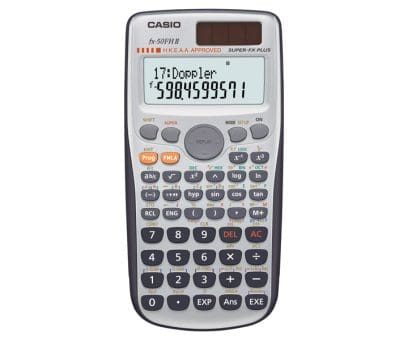Search

HKEAA Approval
Programmable Calculators

# fx-50FHII

My GShock

Favorite## BASIC-like Program, 2-line Display, Multi-replay Function

Program function (4 program areas)
Multi-replay function
2-line big display
Complex number calculations
Combination and permutation
23 built-in formulas
Fraction calculations
40 scientific constants
Statistics (STAT-data editor, Standard deviation, Regression analysis)
7 variables
Base-n calculations/conversions
Logical operations
Comes with slide-on hard case

## Specifications

Product features
Programmable
Non Graphing
Number of Functions : 406
Product type
Programmable
Non Graphing
Number of digits
10 + 2 digits
Liquid crystal display
Dot matrix display
Key characteristics
Plastic keys
Key functions
Negative Sign
Power Off
Memory
Variables: 9 (A,B,C.D.E.F.X.Y,M)
Independent Memory (M / M+ / M-)
Reset Function
Power supply
Solar & Battery
Approximate battery life Main 3 years
Auto Power Off
Accessories
Hard Case
Display format settings
Number Format: Fix
Number Format: Sci
Number Format: Norm
Engineering Notation
Fraction / Decimal Conversion (Fraction Key)
Basic calculation
Basic Calculation
Bracket Calculation
Fraction Calculation
Degree, Minute, Secound (Sexagesimal) Calculations
Reciprocal Calculation
Percent Calculation (scientific calculator function)
Factorial Function
π
Irrational number calcuation
Rounding Function
Transcendental number: e
Base-n calculation
Logical Operators
Complex number calculation
Applied calculation
Multi-statement Command, Output Command (: / ◢)
Continuous Calculation
Replay Function
Multi-replay Function
Basic functions
Basic Mathematical Functions
Number of Functions : 406
Absolute Value Calculation
Power Function (Square)
Power Function (Cube)
Power Function
Power Function (Square Root)
Trigonometric Function
Inverse Trigonometric Function
Hyperbolic / Inverse Hyperbolic Function
Exponential Calculation
Logarithmic Calculation
Log not base 10
Coordinate Conversion
Combination / Permutation
Random Number Generation
Summation
Physics- and chemistry-related functions
Scientific Constants
Statistical data
STAT-data editor
Statistical calculation
Statistics Function
Calculator program function
Programming Function
BASIC LIKE Program
Program capacity
Memory : 680 bytes
Permitted in exams
Hong Kong HKEAA Approval